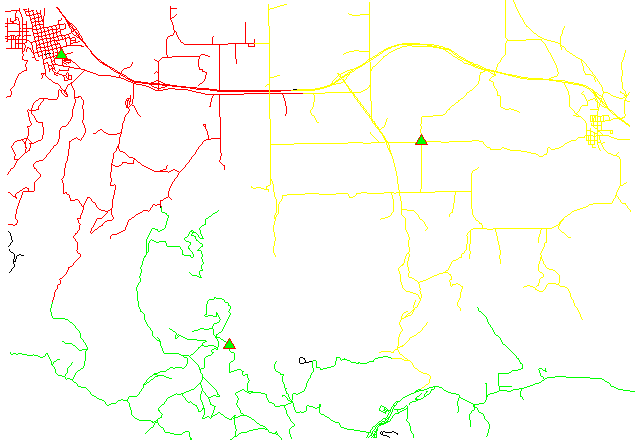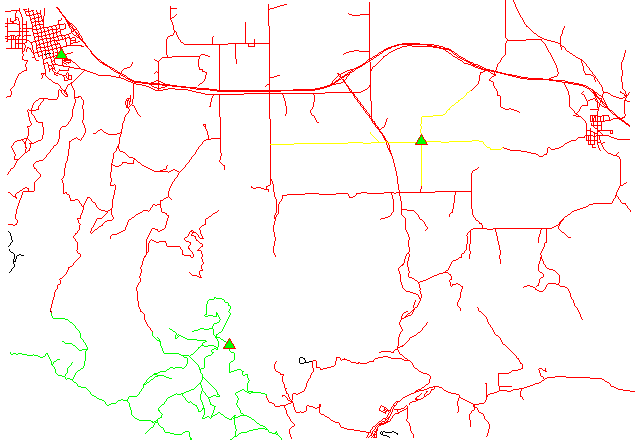Note: A new GRASS GIS stable version has been released: GRASS GIS 7.4, available here.
Updated manual page: here

## NAME

v.net.alloc - Allocates subnets for nearest centers (direction from center).
center node must be opened (costs >= 0). Costs of center node are used in calculation

## KEYWORDS

vector, network, cost allocation

## SYNOPSIS

v.net.alloc
v.net.alloc --help
v.net.alloc [-g] input=name output=name [arc_layer=string] [arc_type=string[,string,...]] [node_layer=string] [arc_column=string] [arc_backward_column=string] [node_column=string] center_cats=range [--overwrite] [--help] [--verbose] [--quiet] [--ui]

### Flags:

-g
Use geodesic calculation for longitude-latitude locations
--overwrite
Allow output files to overwrite existing files
--help
Print usage summary
--verbose
Verbose module output
--quiet
Quiet module output
--ui
Force launching GUI dialog

### Parameters:

input=name [required]
Name of input vector map
Or data source for direct OGR access
output=name [required]
Name for output vector map
arc_layer=string
Arc layer
Vector features can have category values in different layers. This number determines which layer to use. When used with direct OGR access this is the layer name.
Default: 1
arc_type=string[,string,...]
Arc type
Options: line, boundary
Default: line,boundary
node_layer=string
Node layer
Vector features can have category values in different layers. This number determines which layer to use. When used with direct OGR access this is the layer name.
Default: 2
arc_column=string
Arc forward/both direction(s) cost column (number)
arc_backward_column=string
Arc backward direction cost column (number)
node_column=string
Node cost column (number)
center_cats=range [required]
Category values
Categories of centers (points on nodes) to which net will be allocated, layer for this categories is given by nlayer option

## DESCRIPTION

v.net.alloc allocates subnets for nearest centers (direction from center). center nodes must be opened (costs >= 0). Costs of center nodes are used in the calculation.

Costs may be either line lengths, or attributes saved in a database table. These attribute values are taken as costs of whole segments, not as costs to traverse a length unit (e.g. meter) of the segment. For example, if the speed limit is 100 km / h, the cost to traverse a 10 km long road segment must be calculated as
length / speed = 10 km / (100 km/h) = 0.1 h.
Supported are cost assignments for both arcs and nodes, and also different costs for both directions of a vector line. For areas, costs will be calculated along boundary lines.

The input vector needs to be prepared with v.net operation=connect in order to connect points representing center nodes to the network.

## NOTES

Nodes and arcs can be closed using cost = -1.

Center nodes can also be assigned to vector nodes using wxGUI vector digitizer.

## EXAMPLES

1. Subnetwork allocation using distance:2. Subnetwork allocation using traveling time:Example 1: Calculating subnets for 3 center nodes using distances

```# Spearfish

# center nodes:
echo "591235.5|4926306.62|1
596591.8|4917042.5|2
602722.9|4923544.2|3" | v.in.ascii in=- out=centernodes

g.copy vect=roads,myroads

# connect points to network
v.net myroads points=centernodes out=myroads_net op=connect thresh=200

# allocate, specifying range of center cats (easier to catch all):
v.net.alloc myroads_net out=myroads_net_alloc center_cats=1-100000 node_layer=2

# report categories
v.category myroads_net_alloc option=report
```
To display the result, run for example:
```# show result
g.region vector=myroads_net
d.mon x0
d.vect myroads_net layer=1

# the result has to be selected by category number of the relevant node:
d.vect myroads_net_alloc cat=1 col=red layer=1
d.vect myroads_net_alloc cat=2 col=green layer=1
d.vect myroads_net_alloc cat=3 col=yellow layer=1

# center nodes
d.vect myroads_net col=red icon=basic/triangle fcol=green size=12 layer=2
```

Example 2: Calculating subnets for 3 center nodes using traveling time

```# Spearfish

# center nodes:
echo "591235.5|4926306.62|1
596591.8|4917042.5|2
602722.9|4923544.2|3" | v.in.ascii in=- out=centernodes

g.copy vect=roads,myroads

# create lines map connecting points to network
v.net myroads points=centernodes out=myroads_net op=connect thresh=500 arc_layer=1 node_layer=2

# set up costs

# create unique categories for each road in layer 3
v.category in=myroads_net out=myroads_net_time opt=add cat=1 layer=3 type=line

# add new table for layer 3
v.db.addtable myroads_net_time layer=3 col="cat integer,label varchar(43),length double precision,speed double precision,cost double precision,bcost double precision"

# copy road type to layer 3
v.to.db myroads_net_time layer=3 qlayer=1 opt=query qcolumn=label columns=label

# upload road length in miles
v.to.db myroads_net_time layer=3 type=line option=length col=length unit=miles

# set speed limits in miles / hour
v.db.update myroads_net_time layer=3 col=speed val="5.0"
v.db.update myroads_net_time layer=3 col=speed val="75.0" where="label='interstate'"
v.db.update myroads_net_time layer=3 col=speed val="75.0" where="label='primary highway, hard surface'"
v.db.update myroads_net_time layer=3 col=speed val="50.0" where="label='secondary highway, hard surface'"
v.db.update myroads_net_time layer=3 col=speed val="25.0" where="label='light-duty road, improved surface'"
v.db.update myroads_net_time layer=3 col=speed val="5.0" where="label='unimproved road'"

# define traveling costs as traveling time in minutes:

# set forward costs
v.db.update myroads_net_time layer=3 col=cost val="length / speed * 60"
# set backward costs
v.db.update myroads_net_time layer=3 col=bcost val="length / speed * 60"

# subnetwork allocation with fastest paths
v.net.alloc in=myroads_net_time arc_layer=3 node_layer=2 arc_column=cost arc_backward_column=bcost out=myroads_net_alloc_time center_cats=1-3
```
To display the result, run for example:
```# show result
g.region vector=myroads_net
d.mon x0
d.vect myroads_net type=line layer=1

# the result has to be selected by category number of the relevant node:
d.vect myroads_net_alloc_time cat=1 col=red layer=1
d.vect myroads_net_alloc_time cat=2 col=green layer=1
d.vect myroads_net_alloc_time cat=3 col=yellow layer=1

# center nodes
d.vect myroads_net_time col=red icon=basic/triangle fcol=green size=12 type=point layer=2
```

## SEE ALSO

d.path, v.net, v.net.iso, v.net.path, v.net.steiner, v.net.salesman

## AUTHOR

Radim Blazek, ITC-Irst, Trento, Italy
Documentation: Markus Neteler, Markus Metz

Last changed: \$Date: 2014-12-24 06:44:33 -0800 (Wed, 24 Dec 2014) \$

## SOURCE CODE

Available at: v.net.alloc source code (history)

Note: A new GRASS GIS stable version has been released: GRASS GIS 7.4, available here.
Updated manual page: here

© 2003-2018 GRASS Development Team, GRASS GIS 7.0.7svn Reference Manual